Next: Interpretation Up: N narrow slits Previous: N narrow slits

### Analysis

We now use all of the skills we have developed to derive what happens in the case of N identical, very narrow slits, all at equal separations d from one another (Figure 6). The only new technique in this section is that we use the formula for the sum of a geometric series.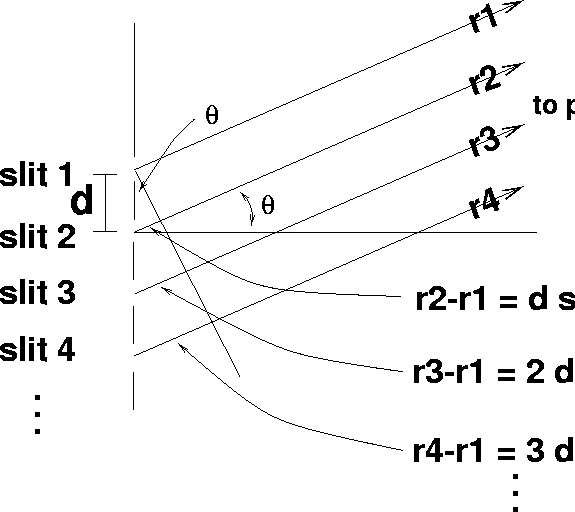Figure 6: Interference experiment with N finite slits

Following our derivation for the two-slit case, we begin with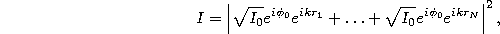where we already have used the fact that all slits let through the same intensity (all I's are equal) and waves arrive at the slits and thus emerge from them all with the same phase (all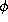's are the same).

Once again, we can factor out the constant phases, and the intensity we would expect from each slit individually, leaving only a sum of exponentials of path differences times k,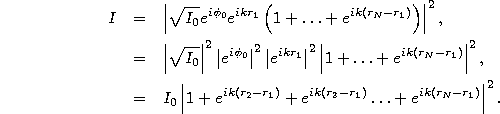The next key step is to realize that the path differences are just multiples of each other. If we define, again,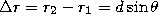, then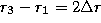,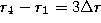,,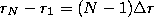. (See Figure 6.) Thus, we must compute the sum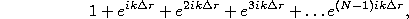but this is just a geometric series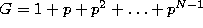with geometric ratio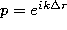. The formula you know for real numbers, G=1/(1-r) still works, and so the sum is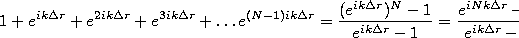To compute the magnitude of this, we use the fact that the magnitude of the ratio is the ratio of the magnitudes. Then, we note that both numerator and denominator have the same form,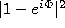, where for the former case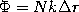and for the latter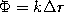. For both cases, we have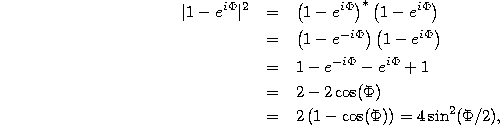where, again, at the last step we use a convenient trig identity. Using this, our final result is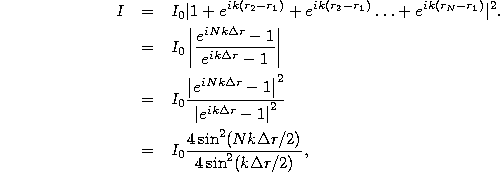which simplifies to our final result,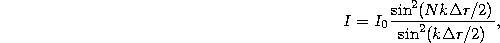where, as before,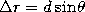.

Tomas Arias
Thu Sep 13 15:26:14 EDT 2001